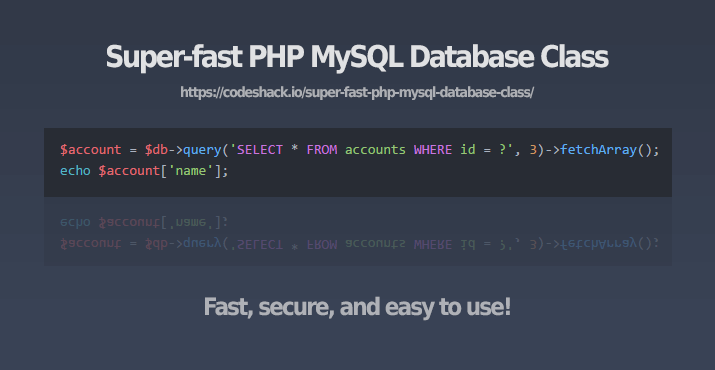# Super-fast PHP MySQL Database ClassThis lightweight database class is written with PHP and uses the MySQLi extension, it uses prepared statements to properly secure your queries, no need to worry about SQL injection attacks.

The MySQLi extension has built-in prepared statements that you can work with, this will prevent SQL injection and prevent your database from being exposed, some developers are confused on how to use these methods correctly so I've created this easy to use database class that'll do the work for you.

This database class is beginner-friendly and easy to implement, with the native MySQLi methods you need to write 3-7 lines of code to retrieve data from a database, with this class you can do it with just 1-2 lines of code, and is much easier to understand.

## Source

Create a new file and name it db.php and add:

PHP Copy
``````<?php
class db {

protected \$connection;
protected \$query;
protected \$show_errors = TRUE;
protected \$query_closed = TRUE;
public \$query_count = 0;

public function __construct(\$dbhost = 'localhost', \$dbuser = 'root', \$dbpass = '', \$dbname = '', \$charset = 'utf8') {
\$this->connection = new mysqli(\$dbhost, \$dbuser, \$dbpass, \$dbname);
if (\$this->connection->connect_error) {
\$this->error('Failed to connect to MySQL - ' . \$this->connection->connect_error);
}
\$this->connection->set_charset(\$charset);
}

public function query(\$query) {
if (!\$this->query_closed) {
\$this->query->close();
}
if (\$this->query = \$this->connection->prepare(\$query)) {
if (func_num_args() > 1) {
\$x = func_get_args();
\$args = array_slice(\$x, 1);
\$types = '';
\$args_ref = array();
foreach (\$args as \$k => &\$arg) {
if (is_array(\$args[\$k])) {
foreach (\$args[\$k] as \$j => &\$a) {
\$types .= \$this->_gettype(\$args[\$k][\$j]);
\$args_ref[] = &\$a;
}
} else {
\$types .= \$this->_gettype(\$args[\$k]);
\$args_ref[] = &\$arg;
}
}
array_unshift(\$args_ref, \$types);
call_user_func_array(array(\$this->query, 'bind_param'), \$args_ref);
}
\$this->query->execute();
if (\$this->query->errno) {
\$this->error('Unable to process MySQL query (check your params) - ' . \$this->query->error);
}
\$this->query_closed = FALSE;
\$this->query_count++;
} else {
\$this->error('Unable to prepare MySQL statement (check your syntax) - ' . \$this->connection->error);
}
return \$this;
}

public function fetchAll(\$callback = null) {
\$params = array();
\$row = array();
while (\$field = \$meta->fetch_field()) {
\$params[] = &\$row[\$field->name];
}
call_user_func_array(array(\$this->query, 'bind_result'), \$params);
\$result = array();
while (\$this->query->fetch()) {
\$r = array();
foreach (\$row as \$key => \$val) {
\$r[\$key] = \$val;
}
if (\$callback != null && is_callable(\$callback)) {
\$value = call_user_func(\$callback, \$r);
if (\$value == 'break') break;
} else {
\$result[] = \$r;
}
}
\$this->query->close();
\$this->query_closed = TRUE;
return \$result;
}

public function fetchArray() {
\$params = array();
\$row = array();
while (\$field = \$meta->fetch_field()) {
\$params[] = &\$row[\$field->name];
}
call_user_func_array(array(\$this->query, 'bind_result'), \$params);
\$result = array();
while (\$this->query->fetch()) {
foreach (\$row as \$key => \$val) {
\$result[\$key] = \$val;
}
}
\$this->query->close();
\$this->query_closed = TRUE;
return \$result;
}

public function close() {
return \$this->connection->close();
}

public function numRows() {
\$this->query->store_result();
return \$this->query->num_rows;
}

public function affectedRows() {
return \$this->query->affected_rows;
}

public function lastInsertID() {
return \$this->connection->insert_id;
}

public function error(\$error) {
if (\$this->show_errors) {
exit(\$error);
}
}

private function _gettype(\$var) {
if (is_string(\$var)) return 's';
if (is_float(\$var)) return 'd';
if (is_int(\$var)) return 'i';
return 'b';
}

}
?>``````

## How To Use

### Connect to MySQL database:

``````include 'db.php';

\$dbhost = 'localhost';
\$dbuser = 'root';
\$dbpass = '';
\$dbname = 'example';

\$db = new db(\$dbhost, \$dbuser, \$dbpass, \$dbname);``````

### Fetch a record from a database:

``````\$account = \$db->query('SELECT * FROM accounts WHERE username = ? AND password = ?', 'test', 'test')->fetchArray();
echo \$account['name'];``````

Or you could do:

``````\$account = \$db->query('SELECT * FROM accounts WHERE username = ? AND password = ?', array('test', 'test'))->fetchArray();
echo \$account['name'];``````

### Fetch multiple records from a database:

``````\$accounts = \$db->query('SELECT * FROM accounts')->fetchAll();

foreach (\$accounts as \$account) {
echo \$account['name'] . '<br>';
}``````

You can specify a callback if you do not want the results being stored in an array (useful for large amounts of data):

``````\$db->query('SELECT * FROM accounts')->fetchAll(function(\$account) {
echo \$account['name'];
});``````

If you need to break the loop you can add:

``return 'break'; ``

### Get the number of rows:

``````\$accounts = \$db->query('SELECT * FROM accounts');
echo \$accounts->numRows();``````

### Get the affected number of rows:

``````\$insert = \$db->query('INSERT INTO accounts (username,password,email,name) VALUES (?,?,?,?)', 'test', 'test', 'test@gmail.com', 'Test');
echo \$insert->affectedRows();``````

### Get the total number of queries:

``echo \$db->query_count;``

### Get the last insert ID:

``echo \$db->lastInsertID();``

### Close the database:

``\$db->close();``

## Conclusion

The database class uses the MySQLi extension, this is built into PHP version >= 5.0.0. If you're using PHP version 5.0.0 to 5.3.0 you'll need install: mysqlnd.

No need to prepare statements using this class, it'll do that for you automatically (write less, do more), your queries will be secure, just remember to make sure you escape your output using htmlspecialchars, or your preferred escaping method.

You're free to use this database class in your projects.